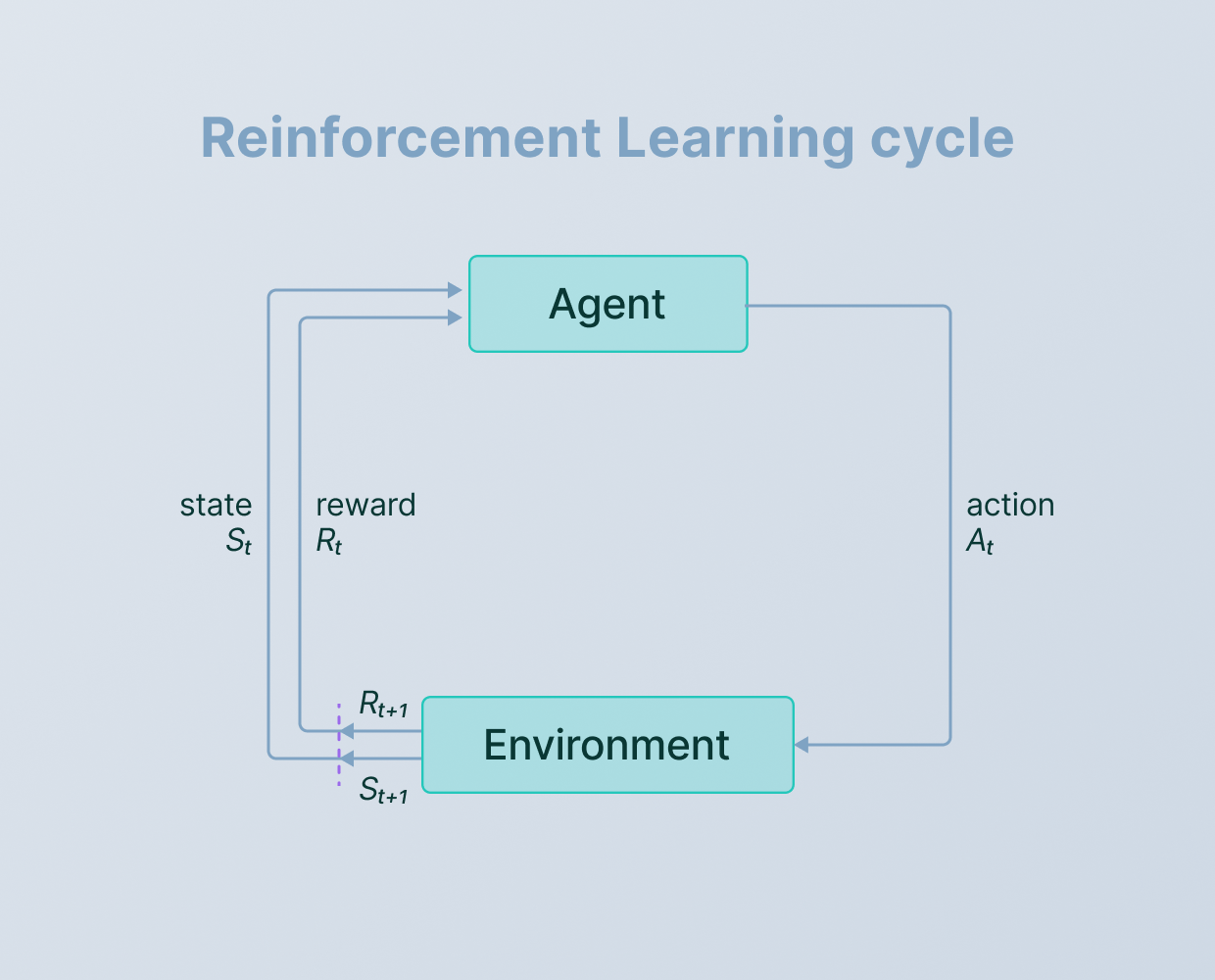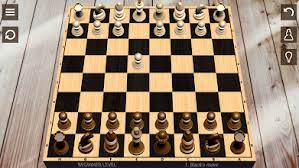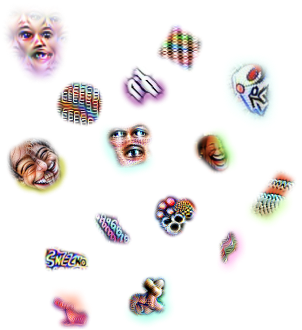# The Beginner's Guide to Deep Reinforcement Learning 

#### The field of deep Reinforcement Learning has literally exploded in the past few years and it found applications in industries such as manufacturing, finance, and healthcare. Read this article to learn how deep Reinforcement Learning works.Reinforcement learning (RL) is a type of machine learning algorithm that falls somewhere between supervised and unsupervised.

It cannot be classified as supervised learning because it doesn't rely solely on a set of labeled training data, but it's also not unsupervised learning because we're looking for our reinforcement learning agent to maximize a reward.

To attain its main goal, the agent must determine the "correct" actions to take in various scenarios.

In this article we’ll explore this concept more in-depth and discuss the following:

Let’s dive in.

## What is deep Reinforcement Learning?

To understand deep Reinforcement Learning better, imagine that you want your computer to play chess with you. The first question to ask is this:

Would it be possible if the machine was trained in a supervised fashion?

In theory, yes. But—

There are two drawbacks that you need to consider.

Firstly, to move forward with supervised learning you need a relevant dataset.

##### 💡 Pro tip: Looking for quality datasets? See 65+ Best Free Datasets for Machine Learning.

Secondly, if we are training the machine to replicate human behavior in the game of chess, the machine would never be better than the human, because it’s simply replicating the same behavior.

So, by definition, we cannot use supervised learning to train the machine.

But is there a way to have an agent play a game entirely by itself?

Yes, that’s where Reinforcement Learning comes into play.

Reinforcement Learning is a type of machine learning algorithm that learns to solve a multi-level problem by trial and error. The machine is trained on real-life scenarios to make a sequence of decisions. It receives either rewards or penalties for the actions it performs. Its goal is to maximize the total reward.

By deep Reinforcement Learning we mean multiple layers of Artificial Neural Networks that are present in the architecture to replicate the working of a human brain.

## Reinforcement Learning definitions

Before we move on, let’s have a look at some of the definitions that you’ll encounter when learning about the Reinforcement Learning.

Agent - Agent (A) takes actions that affect the environment. Citing an example, the machine learning to play chess is the agent.

Action - It is the set of all possible operations/moves the agent can make. The agent makes a decision on which action to take from a set of discrete actions (a).

Environment - All actions that the reinforcement learning agent makes directly affect the environment. Here, the board of chess is the environment. The environment takes the agent's present state and action as information and returns the reward to the agent with a new state.

For example, the move made by the bot will either have a negative/positive effect on the whole game and the arrangement of the board. This will decide the next action and state of the board.

State - A state (S) is a particular situation in which the agent finds itself.This can be the state of the agent at any intermediate time (t).

Reward (R) - The environment gives feedback by which we determine the validity of the agent’s actions in each state. It is crucial in the scenario of Reinforcement Learning where we want the machine to learn all by itself and the only critic that would help it in learning is the feedback/reward it receives.

For example, in a chess game scenario it happens when the bot takes the place of an opponent's piece and later captures it.

Discount factor - Over time, the discount factor modifies the importance of incentives. Given the uncertainty of the future it’s better to add variance to the value estimates. Discount factor helps in reducing the degree to which future rewards affect our value function estimates.

Policy (π) - It decides what action to take in a certain state to maximize the reward.

Value (V) - It measures the optimality of a specific state. It is the expected discounted rewards that the agent collects following the specific policy.

Q-value or action-value - Q Value is a measure of the overall expected reward if the agent (A) is in state (s) and takes action (a), and then plays until the end of the episode according to some policy (π).

## Model-based vs Model-free learning algorithms

There are two main types of Reinforcement Learning algorithms:

1. Model-based algorithms

2. Model-free algorithms

### Model-based algorithms

Model-based algorithm use the transition and reward function to estimate the optimal policy.

• They are used in scenarios where we have complete knowledge of the environment and how it reacts to different actions.
• In Model-based Reinforcement Learning the agent has access to the model of the environment i.e., action required to be performed to go from one state to another, probabilities attached, and corresponding rewards attached.
• They allow the reinforcement learning agent to plan ahead by thinking ahead.
• For static/fixed environments,Model-based Reinforcement Learning is more suitable.

### Model-free algorithms

Model-free algorithms find the optimal policy with very limited knowledge of the dynamics of the environment. They do no thave any transition/reward function to judge the best policy.

• They estimate the optimal policy directly from experience i.e., interaction between agent and environment without having any hint of the reward function.
• Model-free Reinforcement Learning should be applied in scenarios involving incomplete information of the environment.
• In real-world, we don't have a fixed environment. Self-driving cars have a dynamic environment with changing traffic conditions, route diversions etc. In such scenarios, Model-free algorithms outperform other techniques

## Common mathematical and algorithmic frameworks

Now, let’s have a look at some of the most common frameworks used in deep Reinforcement Learning.

### Markov Decision Process (MDP)

Markov Decision Process is a Reinforcement Learning algorithm that gives us a way to formalize sequential decision making.

This formalization is the basis to the problems that are solved by Reinforcement Learning. The components involved in a Markov Decision Process (MDP) is a decision maker called an agent that interacts with the environment it is placed in.

These interactions occur sequentially overtime.

In each timestamp, the agent will get some representation of the environment state. Given this representation, the agent selects an action to make. The environment is then transitioned into some new state and the agent is given a reward as a consequence of its previous action.

Let’s wrap up everything that we have covered till now.

The process of selecting an action from a given state, transitioning to a new state and receiving a reward happens sequentially over and over again. This creates something called a trajectory that shows the sequence of states, actions and rewards.

Throughout the process, it is the responsibility of the reinforcement learning agent to maximize the total amount of rewards that it received from taking actions in given states of environments.

The agent not only wants to maximize the immediate rewards but the cumulative reward it receives in the whole process.

The below image clearly depicts the whole idea.

An important point to note about the Markov Decision Process is that it does not worry about the immediate reward but aims to maximize the total reward of the entire trajectory. Sometimes, it might prefer to get a small reward in the next timestamp to get a higher reward eventually over time.

### Bellman Equations

Let’s cover the important Bellman Concepts before moving forward.

➔ State is a numerical representation of what an agent observes at a particular point in an environment.

➔ Action is the input the agent is giving to the environment based on a policy.

➔ Reward is a feedback signal from the environment to the reinforcement learning agent reflecting how the agent has performed in achieving the goal.

Bellman Equations aim to answer these questions:

The agent is currently in a given state ‘s’. Assuming that we take best possible actions in all subsequent timestamps,what long-term reward the agent can expect?

or

What is the value of the state the agent is currently in?

Bellman Equations are a class of Reinforcement Learning algorithms that are used particularly for deterministic environments.

The value of a given state (s) is determined by taking a maximum of the actions we can take in the state the agent is in.The aim of the agent is to pick the action that is going to maximize the value.

Therefore, it needs to take the addition of the reward of the optimal action ‘a’ in state ‘s' and add a multiplier ‘γ’ that is the discount factor which diminishes its reward over time. Every time the agent takes an action it gets back to the next state ‘s'.

Rather than summing over numerous time steps, this equation simplifies the computation of the value function, allowing us to find the best solution to a complex problem by breaking it down into smaller, recursive subproblems.

### Dynamic Programming

In Bellman Optimality Equations if we have large state spaces, it becomes extremely difficult and close to impossible to solve this system of equations explicitly.

Hence, we shift our approach from recursion to Dynamic Programming.

Dynamic Programming is a method of solving problems by breaking them into simpler sub-problems. In Dynamic Programming, we are going to create a lookup table to estimate the value of each state.

There are two classes of Dynamic Programming:

1. Value Iteration

2. Policy Iteration

### Value iteration

In this method, the optimal policy (optimal action for a given state) is obtained by choosing the action that maximizes optimal state-value function for the given state.

The optimal state-value function is obtained using an iterative function and hence its name - Value Iteration.

By iteratively improving the estimate of V,the Value Iteration method computes the ideal state value function (s). V (s) is initialized with arbitrary random values by the algorithm. The Q (s, a) and V (s) values are updated until they converge. Value Iteration is guaranteed to get you to the best results.

### Policy iteration

This algorithm has two phases in its working:

1. Policy Evaluation - It computes the values for the states in the environment using the policy provided by the policy improvement phase.

2. Policy Improvement - Looking into the state values provided by the policy evaluation part, it improves the policy so that it can get higher state values.

Firstly, the reinforcement learning agents tarts with a random policy π (i). Policy Evaluation will evaluate the value functions like state values for that particular policy.

The policy improvement will improve the policy and give us π (1) and so on until we get the optimal policy where the algorithm stops. This algorithm communicates back and forth between the two phases - Policy Improvement gives the policy to the policy evaluation module which computes values.

Later, looking at the computed policy, policy evaluation improves the policy and iterates this process.

Policy Evaluation is also iterative.

Firstly, the reinforcement learning agent gets the policy from the Policy Improvement phase. In the beginning, this policy is random.

Here,policy is like a table with state-action pairs which we can randomly initialize. Later, Policy Evaluation evaluates the values for all the states. This step goes on a loop until the process converges which is marked by non-changing values.

Then comes the role of Policy Improvement phase. It is just a one-step process. We take the action that maximizes this equation and that becomes the policy for the next iteration.

To understand the Policy Evaluation algorithm better have a look at this.

### Q-learning

Q-Learning combines the policy and value functions,and it tells us jointly how useful a given action is in gaining some future reward.

Quality is assigned to a state-action pair as Q (s,a) based on the future value that it expects given the current state and best possible policy the agent has. Once the agent learns this Q-Function, it looks for the best possible action at a particular state (s) that yields the highest quality.

Once we have an optimal Q-function (Q*), we can determine the optimal policy by applying a Reinforcement Learning algorithm to find an action that maximizes the value for each state.

In other words, Q* gives the largest expected return achievable by any policy π for each possible state-action pair.

In the basic Q-Learning approach, we need to maintain a look-up table called q-map for each state-action pair and the corresponding value associated with it.

Deep Q-Learning aka Deep Q-network employs Neural Network architecture to predict the Q-value for a given state.

## Neural Networks and Deep Reinforcement Learning

Reinforcement Learning involves managing state-action pairs and keeping a track of value (reward) attached to an action to determine the optimum policy.

This method of maintaining a state-action-value table is not possible in real-life scenarios when there are a larger number of possibilities.

Instead of utilizing a table, we can make use of Neural Networks to predict values for actions in a given state.

## Applications of deep Reinforcement Learning

Finally, let’s have a look at some of the real-world applications of Reinforcement Learning.

### Industrial manufacturing

Deep Reinforcement Learning is very commonly applied in Robotics.

The actions that the robot has to take are inherently sequential. Agents learn to interact with dynamic changing environments and thus find applications in industrial automation and manufacturing.

Labor expenses, product faults and unexpected downtime are being reduced with significant improvement in transition times and production speed.

### Self-driving cars

Machine Learning technologies power self-driving cars.

Autonomous vehicle used large amounts of visual data and leveraged image processing capabilities in cohesion with Neural Network architecture.

The algorithms learn to recognize pedestrians,roads, traffic, detect street signs in the environment and act accordingly. It is trained in complex scenarios and trained to excel in decision making skills in scenarios involving minimal human loss, best route to follow etc.

We have seen how supervised learning and time-series analysis helps in prediction of the stock market. But none helps us in making decisions of what to do in a particular situation. An RL agent can select whether to hold, buy, or sell a share. To guarantee that the RL model is working optimally, it is assessed using market benchmark standards.

### Natural Language Processing

Reinforced Learning is expanding in wings and has conquered NLP too. Different NLP tasks like question-answering, summarization, chatbot implementation can be done by a Reinforcement Learning agent.

Virtual Bots are trained to mimic conversations. Sequences with crucial conversation properties including coherence, informativity, and simplicity of response are rewarded using policy gradient approaches.

### Healthcare

Reinforced Learning in healthcare is an area of continuous research. Bots equipped with biological information are extensively trained to perform surgeries that require precision. RL bots help in better diagnosis of diseases and predict the onset of disease if the treatment is delayed and so on.

## Deep Reinforcement Learning: Key Takeaways

Here’s a short recap of everything we’ve learnt about deep Reinforcement Learning so far.

• The essence of Reinforced Learning is to enforce behavior based on the actions performed by the agent. The agent is rewarded if the action positively affects the overall goal.
• The basic aim of Reinforcement Learning is reward maximization. The agent is trained to take the best action to maximize the overall reward.
• RL agents work by using the already known exploited information or exploring unknown information about an environment.
• Policy refers to the strategy the agent follows to determine the next action based on current state.
• In Contrast to Reward, which implies a short-term gain, Value refers to the long-term return with discount.
• The heart of Reinforcement Learning is the mathematical paradigm Markov Decision Process. It is a way of defining the probability of transitioning from one state to another.
• Q-Learning is a model-free based Reinforced Learning algorithm that helps the agent learn the value of an action in a particular state.
• Reinforcement Learning applications include self-driving cars, bots playing games, robots solving various tasks, virtual agents in almost every domain possible.

A Comprehensive Guide to Convolutional Neural Networks

Computer Vision: Everything You Need to Know

What is Data Labeling and How to Do It Efficiently [Tutorial]

Data Cleaning Checklist: How to Prepare Your Machine Learning Data

##### Microsoft

Pragati is a software developer at Microsoft, and a deep learning enthusiast. She writes about the fundamental mathematics behind deep neural networks.

### Upgrade to a new era of software

We're telling the stories of teams that pioneer neural networks to solve any visual task. You can join them by signing up to V7 - the only platform to develop AIs for aony computer vision use case, and monitor them in production.You'll be able to develop your own training data and models, or apply pre-existing AI models to solve new use cases.